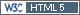## In-vitro Population Bioequivalence [Regulatives / Guidelines]

Hi,

We have questions on In-vitro Population BE study.

The approach used was the proc GLM for calculation MSW_R, MSW_T, MSB_R and MSB_R for parameters of D50 and Span to population bioequivalence.

We use standard algorithm for one life stage (m=1) as defined in draft budesonide guideline recommend sept 2012.

Now problem is our results does not matches with the sponsor results, when we calculated SD_R and SD_T for one life stage (m=1) from Proc GLM model.

There are large differences for the values of SD_R, SD_T and 95% upper confidence bound for linearized criteria of reference-scaled and constant scaled my approach, but the Geometric mean are very similar for both D50 and Span.

They have used the proc mean procedure and directly extracted SD_T and SD_R value and used all remaining calculation.

As mentioned in draft budesonide guideline, for data collected on one life stage (m=1), ignore E2, E4c and E4s and their corresponding H and U terms in the calculation.

Calculation formula for sigmaR and sigmaT (for more than one life stage (m>1)) are as below:

```data FinalRef;            merge both (where=(TRT='R' and _SOURCE_='ERROR') rename=(Mean=Mean_R MS=MSW_R))       both (where=(TRT='R' and _SOURCE_='Container') rename=(MS=MSB_R));     by _name_; run; data FinalTest;              merge both (where=(TRT='T' and _SOURCE_='ERROR') rename=(Mean=Mean_T MS=MSW_T))       both (where=(TRT='T' and _SOURCE_='Container') rename=(MS=MSB_T));     by _name_; run; m_T=1;/* no. of stages-to modify*/ m_R=1;/* no. of stages-to modify*/ n_T=10;/* no. of bottles per batch-to modify*/ n_R=10;/* no. of bottles per batch-to modify*/ l_T=4;/* no. of batches-to modify*/ l_R=4;/* no. of batches-to modify*/ GM_Ratio=exp(mean_T)/exp(mean_R); SD_R=sqrt ((MSB_R/m_R)+(((m_R-1)*MSW_R)/m_R)); SD_T=sqrt ((MSB_T/m_T)+(((m_T-1)*MSW_T)/m_T)); HD=(abs(meandiff)+tval*sqrt((MSB_T/(n_T*l_T*m_T))+(MSB_R/(n_R*l_R*m_R))))**2; UD=(HD-ED)**2; E1=MSB_T/m_T; H1=(l_T*n_T-1)*E1/(cinv(&alpha,(l_T*n_T-1))); U1=(H1-E1)**2; E3s=-(1+&thetap)*MSB_R/m_R; H3s=((l_R*n_R-1)*E3s)/(cinv((1-&alpha),(l_R*n_R-1))); U3S=(H3s-E3s)**2; E3c=-MSB_R/m_R; H3c=(l_R*n_R-1)*E3c/(cinv((1-&alpha),(l_R*n_R-1))); U3c=(H3c-E3c)**2; Eqr=ED+E1+E3s; Hn1=Eqr+sqrt(UD+U1+U3s); Eqc=(ED+E1+E3c-&thetaP*&Sigma2T0); Hn2=Eqc+sqrt(UD+U1+U3c);```

We did not found the algorithm with sigmaR and sigmaT calculation for one life stage (m=1). We have slightly modified SD_R and SD_T formula for one life stage (m=1) and remove (((m_R-1)*MSW_R)/m_R)) and (((m_T-1)*MSW_T)/m_T)) from above equation.

Modified equation are as below:
```SD_R=sqrt (MSB_R/m_R) SD_T=sqrt (MSB_T/m_T)```

Here we are confuse with approach which is based on the draft guideline.

Can anybody comment of formula on SD_R and SD_T calculation for one life stage (m=1)?

Kumar NaiduIng. Helmut Schütz